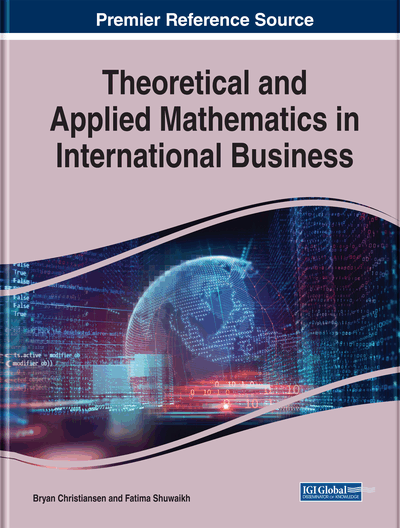# The PSK Method for Solving Fully Intuitionistic Fuzzy Assignment Problems With Some Software Tools

P. Senthil Kumar (Navodaya Institute of Technology, India)
DOI: 10.4018/978-1-5225-8458-2.ch009

## Abstract

The assignment problem (AP) is a particular case of a linear programming problem that deals with the allocation of various resources for various activities on a 1-to-1 basis. It does so in such a manner that the profit or sale involved in the process is maximum and cost or time is minimum. Generally, the profit/sale/cost/time is called the parameter of the AP and this is not a crisp number due to some uncontrollable factors. They can also involve uncertainty and hesitation. Therefore, to solve the AP under an intuitionistic fuzzy environment in this chapter, the author proposes the PSK (P. Senthil Kumar) method. Numerous theorems which are related to intuitionistic fuzzy assignment problem is proposed and is proved by PSK. By using the PSK method, the real-life related fully intuitionistic fuzzy assignment problems are solved. The proposed results are verified by both LINGO 17.0 and TORA software packages. In addition to verifying the efficiency and realism of the proposed method, the computer code based on LINGO 17.0 is presented. Results, discussion, comparative study, and the advantages of the PSK method are given. The chapter ends with the conclusion and future studies.
Chapter Preview
Top

## Introduction And Literature Review

The Assignment Problem (AP) is used worldwide in solving real-world problems. An AP plays an important role in assigning drivers to trucks, trucks to routes, persons to jobs, operators to machines, or problems to research teams. The AP is a special case of the linear programming problem (LPP) in which the aim of the decision maker (DM) is to assign n number of jobs to n number of machines (persons) at a minimum cost/minimum time/maximum profit. To find the solution to APs in the extant literature, Kuhn (1955) proposed the Hungarian method while Thompson (1981) discussed a recursive method for solving the AP. Avis and Devroye (1985) presented an analysis of a decomposition heuristic for the AP and Balinski (1986) developed a competitive (dual) simplex method. Paparrizos (1991) created an efficient exterior point simplex type algorithm for the AP. Barr et al. (1977) gave the alternating basis algorithm for assignment problems.

Ping et al. (1997) discussed a new algorithm for the AP which they also called an alternative to the Hungarian Method. Their assignment algorithm is based on a 2n*2n matrix in which operators performed on the matrix until an optimal solution is found. Lin and Wen (2004) proposed an efficient algorithm based on a labeling method for solving the linear fractional programming case. Singh (2012) discussed note on assignment algorithms with easy method of drawing lines to cover all zeros. However, in real-life situations, the parameter of the AP is in imprecise instead of fixed real numbers because the time/cost/profit for completing a job by a facility (machine/person) might vary due to different situations.

To deal quantitatively with imprecise information in making decisions, Zadeh (1965) introduced the fuzzy set theory and has applied it successfully in various fields. The use of fuzzy set theory increased dramatically in the field of optimization after the pioneering work by Bellman and Zadeh (1970). The fuzzy set deals with the degree of membership (belongingness) of an element in the set, but it does not consider the non-membership (non-belongingness) of an element in the set. In a fuzzy set, the membership value (level of acceptance or level of satisfaction) lies between 0 and 1, whereas in a crisp set the element belongs to the set that represents 1 and the element not in the set that represents 0.

Therefore, the applications of fuzzy set theory enabled many practitioners to solve assignment, transportation, and LPPs by using fuzzy representation for data. Kumar et al. (2009) proposed a method for solving fully fuzzy assignment problems by using triangular fuzzy numbers. Mohideen and Kumar (2010) presented a comparative study on transportation problem in fuzzy environments. Mukherjee and Basu (2010) presented an application of fuzzy ranking method for solving assignment problems with fuzzy costs. Kumar and Gupta (2012) investigated assignment and travelling salesman problems with cost coefficients as LR fuzzy parameters.

## Complete Chapter List

Search this Book:
Reset